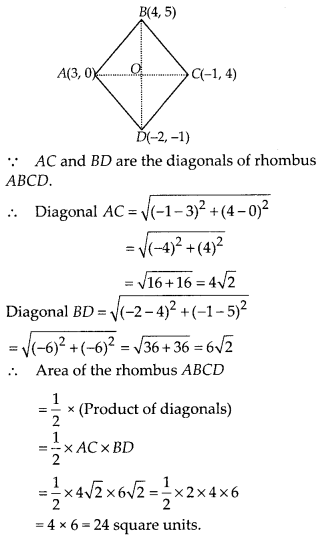# MP Board Class 10th Maths Solutions Chapter 7 Coordinate Geometry Ex 7.2

In this article, we will share MP Board Class 10th Maths Book Solutions Chapter 7 Coordinate Geometry Ex 7.2 Pdf, These solutions are solved subject experts from the latest edition books.

## MP Board Class 10th Maths Solutions Chapter 7 Coordinate Geometry Ex 7.2

Question 1.
Find the coordinates of the point which divides the join of (-1, 7) and (4, -3) in the ratio 2 : 3.
Solution:
Let the required point be P(x, y).
Here the end points are (-1, 7) and (4, – 3)
Ratio = 2 : 3 = m1 : m2
∴ x = $$\frac{m_{1} x_{2}+m_{2} x_{1}}{m_{1}+m_{2}}$$
= $$\frac{(2 \times 4)+3 \times(-1)}{2+3}=\frac{8-3}{5}=\frac{5}{5}=1$$
And y = $$\frac{m_{1} y_{2}+m_{2} y_{1}}{m_{1}+m_{2}}$$
= $$\frac{2 \times(-3)+(3 \times 7)}{2+3}=\frac{-6+21}{5}=\frac{15}{5}=3$$
Thus, the required point is (1, 3)

Question 2.
Find the coordinates of the points of trisection of the line segment joining (4, -1) and (-2, -3).
Solution:
Let the given points be A(4, -1) and B(-2, -3)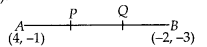Let the points P and Q trisects AB.
i.e., AP = PQ = QB
i.e., P divides AB in the ratio of 1 : 2 and Q divides AB in the ratio of 2 : 1
Let the coordinates of P be (x, y)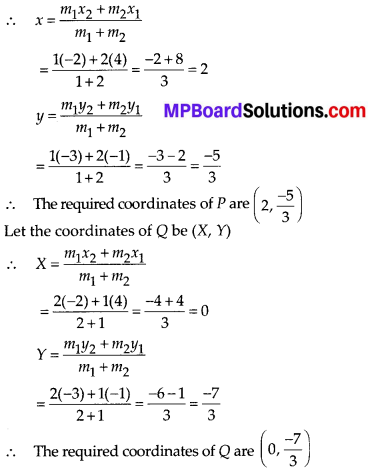Question 3.
To conduct Sports Day activities, in your rectangular shaped school ground ABCD, lines have been drawn with chalk powder at a distance of 1 m each. 100 flower pots have been placed at a distance of 1 m from each other along AD, as shown in the figure. Niharika runs 1/4th the distance AD on the 2nd line and posts a green flag. Preet runs 1/5th the distance AD on the eighth line and posts a red flag. What is the distance between both the flags? If Rashmi has to post a blue flag exactly halfway between the line segment joining the two flags, where should she post her flag ?.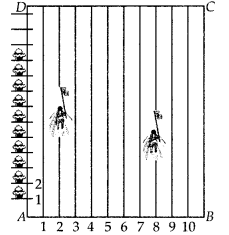Solution:
Let us consider ‘A’ as origin, then
AB is the x-axis.
Now, the position of green flag-post is (2, $$\frac{100}{4}$$) or (2, 25)
And the position of the red flag-post is (8, $$\frac{100}{5}$$) or (8, 20)
Distance between both the flags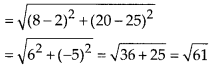Let the mid-point of the line segment joining the two flags be M(x, y).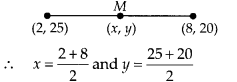or x = 5 and y = 22.5
Thus, the blue flag is on the 5th line at a distance 22-5 m above AB.

Question 4.
Find the ratio in which the line segment joining the points (-3, 10) and (6, -8) is divided by (-1, 6).
Solution:
Let the given points are A(-3, 10) and B(6, -8).
Let the point P(-1, 6) divides AB in the ratio m1 : m2.
∴ Using the section formula, we have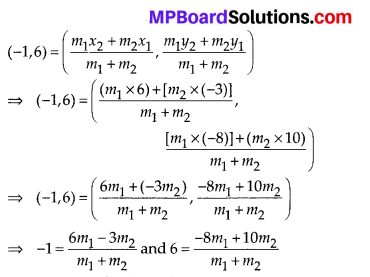⇒ -1(m1 + m2) = 6m1 – 3m2
and 6(m1 + m2) = – 8m1 + 10m2
⇒ -m1 – m2 – 6m1 + 3m2 = 0
and 6m1 + 6m2 + 8m1 – 10m2 = 0
⇒ -7m1 + 2m2 = 0 and 14m1 – 4m2 = 0 or 7m1 – 1m2 = 0
⇒ $$\frac{m_{1}}{m_{2}}=\frac{2}{7}$$ and $$\frac{m_{1}}{m_{2}}=\frac{2}{7}$$
⇒ 2m2 = 7m1 and 7m1 = 2m2
⇒ m1 : m2 = 2 : 7 and m1 : m2 = 2 : 7
Thus, the required ratio is 2 : 7.Question 5.
Find the ratio in which the line segment joining 4(1, -5) and B(-4, 5) is divided by the x-axis. Also, find the coordinates of the point of division.
Solution:
The given points are A( 1, -5) and B(-4, 5). Let the required ratio = k:l and the required point be P(x, y).
Since the point P lies on x-axis,
∴ Its y-coordinate is 0.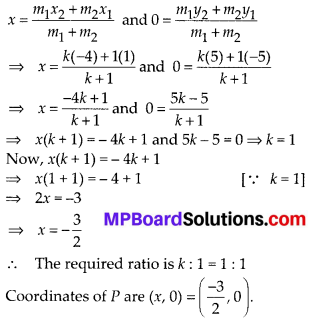Question 6.
If (1, 2), (4, y), (x, 6) and (3,5) are the vertices of a parallelogram taken in order, find x and y.
Solution:
Let the given points are A( 1, 2), B(4, y), C(x, 6) and D(3, 5)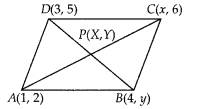Since, the diagonals of a parallelogram bisect each other.
∴ The coordinates of P are :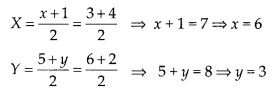∴ The required values of x and y are 6 and 3 respectively.

Question 7.
Find the coordinates of a point A, where AB is the diameter of a circle whose centre is (2, -3) and Bis (1,4).
Solution:
Let centre of the circle is 0(2, -3) and the end points of the diameter be A(x, y) and B(1, 4)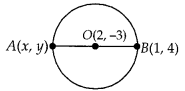Since, the centre of a circle bisects the diameter.
∴ 2 = $$\frac{x+1}{2}$$ ⇒ x + 1 = 4 or x = 3
And -3 = $$\frac{y+4}{2}$$ ⇒ y + 4 = -6 or y = -10
Thus, the coordinates of A are (3, -10)Question 8.
If A and B are (-2, -2) and (2, -4) respectively, find the coordinates of P such that AP = $$\frac{3}{7}$$ AB and Plies on the line segment AB.
Solution:
Here, the given points are A(-2, -2) and B(2, -4)
Let the coordinates of P are (x, y)
Since, the point P divides AB such that
AP = $$\frac{3}{7}$$AB or $$\frac{A P}{A B}$$ = $$\frac{3}{7}$$
⇒ AB = AP + BP
⇒ $$\frac{A P+B P}{A P}$$ = $$\frac{7}{3}$$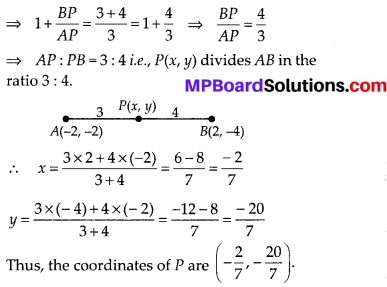Question 9.
Find the coordinates of the points which divide the line segment joining A(-2, 2) and B(2, 8) into four equal parts.
Solution:
Here, the given points are A(-2, 2) and B(2, 8)
Let P1, P2 and P3 divide AB in four equal parts.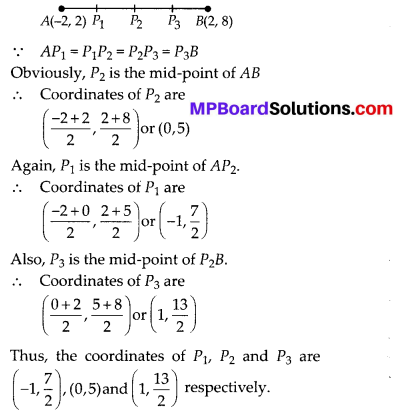Question 10.
Find the area of a rhombus if its vertices are (3, 0), (4, 5), (-1, 4) and (-2, -1) taken in order.
[Hint: Area of a rhombus = $$\frac{1}{2}$$ (product of its diagonals)]
Solution:
Let the vertices of the given rhombus are A(3, 0), B(4, 5), C(-1, 4) and D(-2, -1)